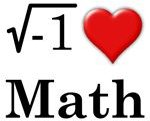# The idea of a proof

In an earlier post I talked about the notions of a lemma, a theorem, a proposition and a corollary. There is in truth, not much difference. So, now I want to say a few informal words about the notion of a proof.

Proof
A proof is a demonstration that if some statements are true, or assumed to be true (i.e. axioms), then some other mathematical statement or statements are necessarily true.

A proof is based on deductive reasoning; if “something” then “something”. All proofs employ logic, though most proofs in mathematics will employ informal logic, rather than formal logic which is the subject of proof theory, a subject I know little about.

The rigor and style of a proof will vary mathematician to mathematician as well as subject to subject. The main thing is if you produce a proof of some statement in some area of mathematics then it should be of a style and at the level of rigor accepted by the mathematicians working in that area.

The key thing about a proof is that it should cover all cases as specified by the statement. “Proofs” that establish the statement for only a fraction of the possible cases will not establish the statement for all cases.

Once a statement is proved it is rather “cast in stone”. It is an unshakable fact that is absolutely true now, as well as always having been true and will always be true.

That all said, mathematicians are human and mistakes can creep in as well as loopholes due to the level of rigor. Worse than that we have Gödel’s incompleteness theorems, but lets forget all about that and another possible complications.

Inductive reasoning
Proofs of statements follow from previously established statements or statements taken to be true.

Discovering a new statement does not always follow in a deductive fashion from earlier statements, though the full proof will. Often the reasoning is more inductive following the creation of specific examples and weakening restrictions on earlier statements.

The discovery of new mathematics can be inductive, but the presentation of new mathematics is usually deductive.

Examples
Finding examples to show that a statement is true, is in my opinion quite important. Also counter examples can be very illustrative. One can certainly build a picture of what is going on that way and build confidence in ones ideas, but only an exhaustive presentation of all the possible cases would constitute a proof. In practice this can simply be impossible; for example one could not prove a theorem on prime numbers that way as there is an infinite number of prime numbers!

An example where an exhaustive exploration of all possible cases is possible is the four colour theorem which has 1,936 cases. The theorem was proved in 1976 by Kenneth Appel and Wolfgang Haken using a computer. There proof is the first proof constructed using a computer that has been generally accepted.

Constructive and nonconstructive proofs
A proof of some statement may just constitute the establishment that a required mathematical object exists. The proof may not give you any indication about how to construct such an object: these proofs are usually to do with “pure existence theorems”.

This is the difference between constructive and nonconstructive proofs. The first gives you the required object explicitly, while the latter only establishes that such an object exists.

One can then argue how “useful” a nonconstructive proof is if you cannot actually find the object required. That said, one may only really need to know that it exists to establish further mathematics.

A proof should be illustrative
Hopefully you have a watertight proof of some statement. That is the main thing, however a proof should also make it clear why the statement is true. Not only will this make your statement clearer, but also it might allow further generalisations of your statement very directly.

Final remarks
There is a lot more to proofs than I have said here, there is a whole branch of logic devoted to the notion of proof. For most working mathematicians proof theory, as it is known, would be “overkill”. Most proofs are, in accordance with proof theory, informal proofs which employ some logic and natural language, but not the full machinery of logic.Public domain image by Peter Valberg It is probably worth comparing mathematics with the empirical sciences with the notion of a proof in mind, but that is a post for another time.